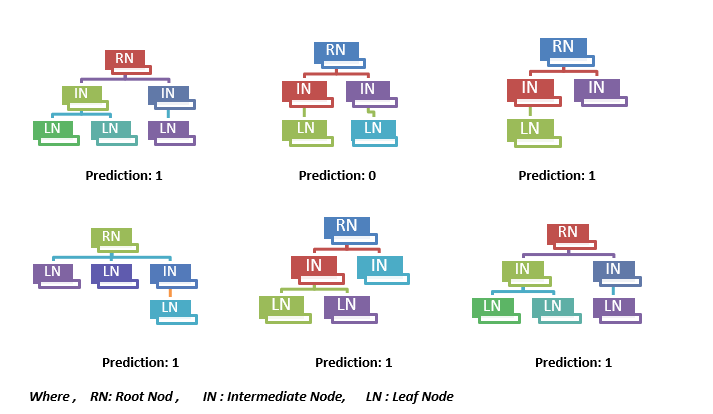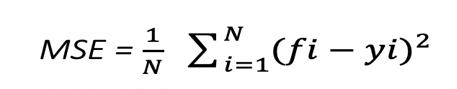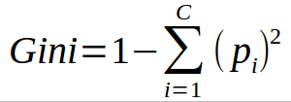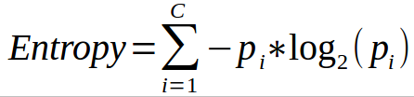### Random Forest Tutorial for Beginners

• date 15th February, 2020 |
• by Prwatech |

# Random Forest Tutorial for Beginners

Random Forest Tutorial for Beginners, Are you the one who is looking forward to knowing about the random forest in Machine Learning? Or the one who is looking forward to knowing why the random forest is better and the advantages and disadvantages of random forest or Are you dreaming to become to certified Pro Machine Learning Engineer or Data Scientist, then stop just dreaming, get your Data Science certification course with Machine Learning from India’s Leading Data Science training institute.

The Random forest is a supervised algorithm and can be used for both classifications as well as regression type of problems in Machine Learning. It handless non-linearity by exploiting correlation in between data points. In this blog, we will learn How the random forest algorithm works in Machine Learning and. Do you want to know random forest introduction and why the random forest is better, So follow the below mentioned random forest tutorial for beginners from Prwatech and take advanced Data Science training with Machine Learning like a pro from today itself under 10+ Years of hands-on experienced Professionals.

## Random Forest in Machine Learning

Classification is an important methodology that lies in supervised learning, which helps to classify the objects or data points of different properties. A precise classification is needed for classification based on different features is a key point in various business fields. Data science has the provision of many algorithms for this like logistic regression, naive Bayes classifier, and support vector machine and decision trees. But the most popular technique is Random Forest.

The Random forest is nothing but a combination of decisions to identify and locate the data point, inappropriate class. Before moving towards Random forest let’s revise the Decision Tree algorithm.

### Decision Tree-basic building block for the random forest:

The decision tree is the most influential and popular tool for classification and prediction. Let’s see one example. Let’s consider we have a dataset having some objects. For classifying that we have to ask some questions.Although we saw the classification method for a very simple example, the logic for the classification remains the same for real-time datasets. But for the real-time larger data sets, it will be more beneficial to classify the objects using different decision trees performing collectively to give a more efficient prediction. Here comes the concept of Random Forest.

## Random Forest Introduction:

The Random forest is nothing but a set of a large number of discrete decision trees that work collaboratively. Each individual tree in the random forest gives a class prediction and the class with the most votes becomes the model’s final prediction. For example, a Random forest has 6 decision trees giving results as follows:Most of the decision trees are giving output prediction as ‘1’. So, the overall prediction output in this random forest will be ‘1’. So random forest is said to be a large number of relatively uncorrelated trees operating as a committee, which will beat any of the individual integral models. Here the key is a low correlation between trees. Uncorrelated models can generate collaborative predictions that are more precise than any of the individual predictions.

The trees prevent individual errors as long as they don’t constantly give errors in the same direction. So, the prerequisites for random forest include some signal in features so that models built using those features do better than random guessing. The predictions and errors made by the individual trees need to have low correlations with each other. Hence chances of getting appropriate predictions are more.

The random forest can be used for regression and classification problems, making it a miscellaneous model.

Prevents the overfitting of data.

Fast to train with test data.

The process of predictions becomes slow, once the model is made.

The presence of outliers can impact overall performance.

### Why Random Forest is Better?

#### Regression Problems:

We have to use the mean squared error (MSE), while applying a random forest algorithm to see how your data divide from each node.Where

N: Number of data points

fi: The value returned by the model

Yi: The actual value for data point i

The above formula measures the distance of each node from the predicted actual value, helps to decide which branch has the better decision for your forest. ‘yi’ is the value of data point that is tested at a specific node and ‘fi’ is the returned value, by a decision tree.

### Classification Problems:

While performing Random Forests based on a classification dataset, you must know that you are normally using the Gini index, or the formula used to decide how nodes on a decision tree branch.In this formula the class and probability to determine the Gini of each branch on a node, determining which branches are more likely to occur. Here, pi represents the relative frequency of a class you are observing in the dataset and c represents the number of classes. You can even use entropy to determine how nodes branch in a decision tree.Entropy uses the probability of a certain outcome to make a decision on how a node should branch.

### How Random Forest Algorithm works in Machine Learning?

Using python we can implement random forest as follows:

### Initialize and import Libraries

import numpy as np

import pandas as pd

import matplotlib.pyplot as plt

### Divide the data set into independent and dependent parts and perform feature selection

x = data.iloc[:,0:20]  #independent columns

y = data.iloc[:,-1]     #dependent columns

### Split the dataset into two parts for training and testing

from sklearn.model_selection import train_test_split

X_train, X_test, y_train, y_test = train_test_split(X, y, random_state = 47, test_size = 0.33)

### Import the Random Forest model from sklearn and build a random forest model.

from sklearn.ensemble import RandomForestClassifier

clf = RandomForestClassifier(n_jobs=2, random_state=0)

### Fit the training data set of dependent and independent values

clf.fit(X_train, y_train)

### Predict the output as per the trained model

preds = clf.predict(X_test)

### Check the Accuracy of the Model (More the value close to 100% better the model)

metrics.accuracy_score(y_test, y_pred)*100)

We hope you understand the random forest tutorial for beginners. Get success in your career as a Data Scientist by being a part of the Prwatech, India’s leading Data Science training institute in Bangalore.

### Quick Support